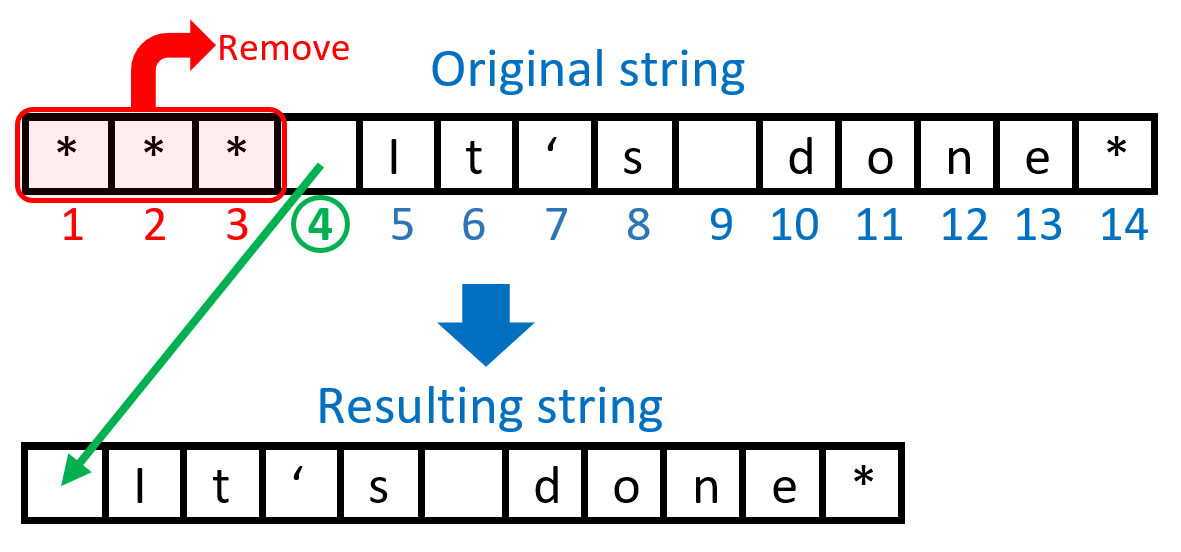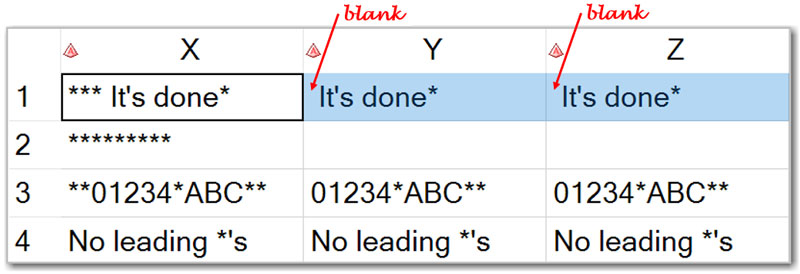As in many other programming languages, there is a very useful SAS function that removes leading blanks in character strings. It is the ubiquitous LEFT function.

The LEFT(x) function left-aligns a character string x, which effectively removes leading blanks.

However, in many SAS applications we need a similar but more versatile data cleansing functionality allowing for removal of other leading characters, not just blanks. For example, consider some bank account numbers that are stored as the following character strings:

123456789
0123456789
000123456789

These strings represent the same account number recorded with either no, one, or several leading zeros. One way of standardizing this data is by removing the leading 0's. And while we're at it, why don’t we address the leading character removal functionality for any leading characters, not just zeros.

## How to remove any leading characters

For example, let’s remove all occurrences of the arbitrary leading character '*'. The following diagram illustrates what we are going to achieve and how:In order to remove a specified character (in this example '*') from all leading positions in a string, we need to search our string from left to right and find the position of the first character in that string that is not equal to the specified character. In this case, it’s a blank character in position 4. Then we can extract a substring starting from that position till the end of the string.

I can see two possible solutions.

#### Solution 1: Using VERIFY() function

The VERIFY (X, C) function searches string X from left to right and returns the position P of the first character that does not appear in the value of C.

Then we can apply the SUBSTR(X,P) function that extracts a substring of X starting from position P till the end of the string X.

#### Solution 2: Using FINDC() function

The FINDC(X, C, ‘K’) function also searches string X from left to right and returns the position P of the first character that does not appear in C. (The modifier ‘K’ switches the default behavior of searching for any character that appears in C to searching for any character that does not appear in C.)

Then, as with the VERIFY() function, we can apply the SUBSTR(X,P) function that extracts a substring of X starting from position P till the end of the string X.

## Special considerations

So far so good, and everything will be just hunky-dory, right? Not really - unless we cover our bases by handling edge cases.

Have we thought of what would happen if our string X consisted of all '*' characters and nothing else? In this special case, both the verify() function and findc() function will find no position of the character that is not equal to '*' and thus return 0.

However, 0 is not a valid second argument value for the SUBSTR(X,P) function. Valid values are 1 . . . through length(X). Having a 0 value for the second argument will trigger the automatic data step variable _ERROR_=1 and the following note generated in the SAS log:

NOTE: Invalid second argument to function SUBSTR at line ## column #.

Therefore, we need to handle this special case separately, conditionally using SUBSTR(X,P) for P>0 and assigning blank ('') otherwise.

## Code implementation for removing leading characters

Let’s put everything together. First, we'll create a test data table:

```data TEST; input X \$ 1-20; datalines; *** It's done* ********* **01234*ABC** No leading *'s ;```

Then we apply the logic described above. The following DATA step illustrates our two implemented coding solutions for removing leading characters:

```data CLEAN (keep=X Y Z); set TEST; C = '*'; *<- leading character(s) to be removed;   P1 = verify(X,C); *<- Solution 1; if P1 then Y = substr(X, P1); else Y = '';   P2 = findc(X,C,'K'); *<- Solution 2; if P2 then Z = substr(X, P2); else Z = '';   put _n_= / X= / P1= / Y= / P2= / Z= /; run;```

Alternatively, we can replace the IF-THEN-ELSE construct with this IFC() function one-liner:

```data CLEAN (keep=X Y Z); set TEST; C='*'; *<- leading character(s) to be removed;   P1 = verify(X,C); *<- Solution 1; Y = ifc(P1, substr(X, P1), '');   P2 = findc(X,C,'K'); *<- Solution 2; Z = ifc(P2, substr(X, P2), '');   put _n_= / X= / P1= / Y= / P2= / Z= /; run;```

The SAS log will show interim and final results by the DATA step iterations:

```_N_=1 X=*** It's done* P1=4 Y=It's done* P2=4 Z=It's done*   _N_=2 X=********* P1=10 Y= P2=10 Z=   _N_=3 X=**01234*ABC** P1=3 Y=01234*ABC** P2=3 Z=01234*ABC**   _N_=4 X=No leading *'s P1=1 Y=No leading *'s P2=1 Z=No leading *'s```

Here is the output data table CLEAN showing the original string X, and resulting strings Y (solution 1) and Z (solution 2) side by side:As you can see, both solutions (1 & 2) produce identical results.

## Conclusion

Compared to the LEFT() function, the solution presented in this blog post not only expands leading character removal/cleansing functionality beyond the blank character exclusively. Using this coding technique we can simultaneously remove a variety of leading characters (including but not limited to blank). For example, if we have a string X=' 0.000 12345' and specify C = ' 0.' (the order of characters listed within the value of C does not matter), then all three characters ' ', '0', and '.' will be removed from all leading positions of X. The resulting string will be Y='12345'.